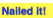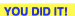# Climbing Alpine Peaks

## OBJECTIVE: To learn how to graph a line on the Cartesian plane using a point and the slope

In a previous lesson, you learned how to graph a line on the Cartesian plane using the slope and the y-intercept. Riding the Berms: ﻿https://www.geogebra.org/m/nkwt8g3y﻿ In this lesson, you'll be introduced to yet another form of the equation of a line—the POINT-SLOPE FORM, (y - y₁) = m(x - x₁) where (x₁, y₁) are the coordinates of a point and m is the slope. The process of graphing a line using a point and the slope is very similar to graphing a line using the slope and the y-intercept. In fact, the y-intercept is just another point, but it is a special point in the sense that it always lies on the y-axis. Interact with the applet below to learn how to graph a line using a point (any point) and the slope.

## INSTRUCTIONS:

1. Move point A to the position indicated by (x₁, y₁) in the linear equation. 2. Use rise/run to locate point B on the line. 3. If your graph is correct, the expressionwill appear. Otherwise, you'll have to try again. 4. Click "Generate New Line" to come up with another problem. 5. Repeat as many times as needed to master the concept.
The applet below shows a variation of the POINT-SLOPE FORM, y = m(x - x₁) + y₁ where (x₁, y₁) are the coordinates of a point and m is the slope. Interact with the applet to learn how to graph a line using a point (any point) and the slope.

## INSTRUCTIONS:

1. Move point A to the position indicated by (x₁, y₁) in the linear equation. 2. Use rise/run to locate point B on the line. 3. If your graph is correct, the expressionwill appear. Otherwise, you'll have to try again. 4. Click "Generate New Line" to come up with another problem. 5. Repeat as many times as needed to master the concept.

QUESTION: In getting the coordinates (x₁, y₁) in the second formula, what do you observe about the sign of y₁?

## TODAY you learned how to graph a line on the Cartesian plane using a point and the slope.

In future lessons, you'll learn how to work out the equations of a line from the graphs. Did you ENJOY your lesson today?